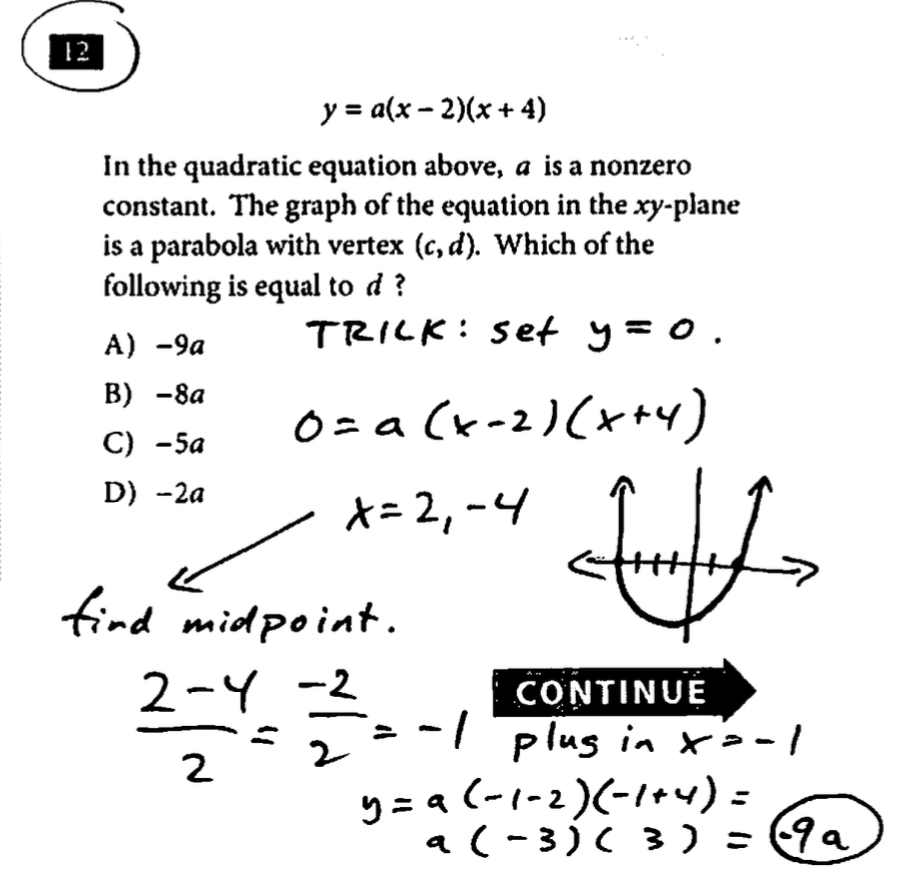# SAT Math Tips Post

## SAT Question of the Day: Test #3, Sec 3 Math NC #12

y = a (x - 2)(x + 4)

"In the quadratic equation above, a is a nonzero constant. The graph of the equation in the xy-plane is a parabola with vertex (c, d).  Which of the following is equal to d ?"

A) -9a
B) -8a
C) -5a
D) -2a

The answer is Choice A, -9a.  Attached is a visual that should help.